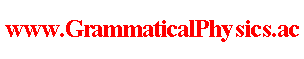since 2006
Help　Sitemap
< Forum >
< Products >
< Quantum History Theory >
< Exact Solution of Uda Equation (1) >

On 2015/12/18, I discovered that the Uda equation has the following solution when V =0.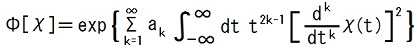where ak is determined by some appropriate condition.

I will prove it below.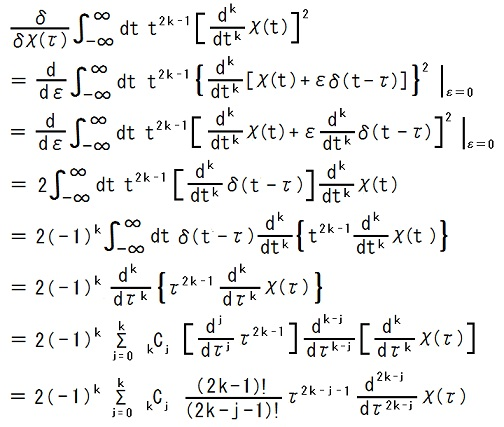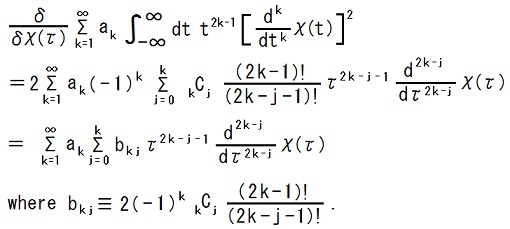j 0 1 2 3 4 5 6 ・・・ k 1 2k-j =2 2k-j =1 2 2k-j =4 2k-j =3 2k-j =2 3 2k-j =6 2k-j =5 2k-j =4 2k-j =3 4 2k-j =8 2k-j =7 2k-j =6 2k-j =5 2k-j =4 5 2k-j =10 2k-j =9 2k-j =8 2k-j =7 2k-j =6 2k-j =5 6 2k-j =12 2k-j =11 2k-j =10 2k-j =9 2k-j =8 2k-j =7 2k-j =6 ・ ・ ・ ・ ・ ・ ・ ・ ・ ・ ・ ・ ・ ・ ・ ・ ・ ・ ・ ・ ・ ・ ・ ・ ・ 　　・ 　　　　・

 2k-j = 2s-1 (s∈N) (k, j) = (s, 1), (s + 1, 3), (s + 2, 5), ・・・, (2s - 1, 2s - 1) s ≦ k ≦ 2s - 1 j = 2(k - s) + 1 2k-j = 2s (s∈N) (k, j) = (s, 0), (s + 1, 2), (s + 2, 4), ・・・, (2s, 2s) s ≦ k ≦ 2s j = 2(k - s)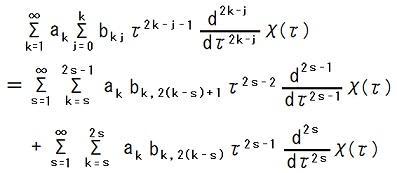I want to adjust the value of ak such that all terms other than the term of 2k - j = 1 vanishes.

Σk=s2s-1 ak bk, 2(k-s)+1 = 0 (s≧2)
Σk=s2s ak bk, 2(k-s) = 0 (s∈N)

∴ a2s-1 = -(1/b2s-1, 2s-1k=s2s-2 ak bk, 2(k-s)+1 (s≧2)
a2s = -(1/b2s, 2sk=s2s-1 ak bk, 2(k-s) (s∈N)

a2 = -(1/b22)a1b10
= (1/6)a1 ∵ b22 = 12, b10 = -2,
a3 = -(1/b33)a2b21
= (1/10)a2 ∵ b33 = -120, b21 = 12
= (1/60)a1,
and so on.

After the adjustment, the following condition holds.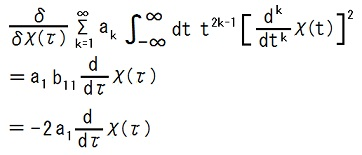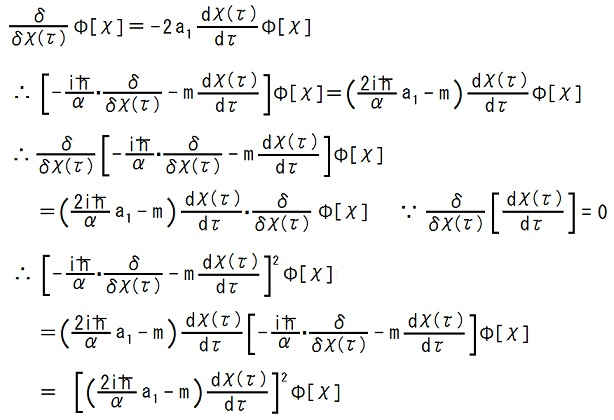When a1 = mα/(ih), it holds that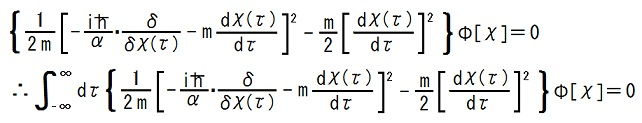.
This is the same as
the reduced form of the Uda equation when V = 0.

a2 = (1/6)a1 = mα/(6ih),
a3 = (1/60)a1 = mα/(60ih),
and so on.

---